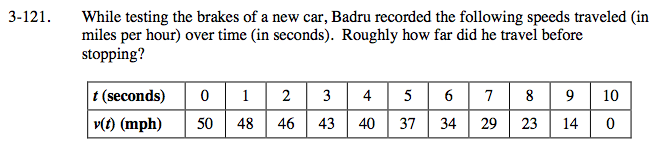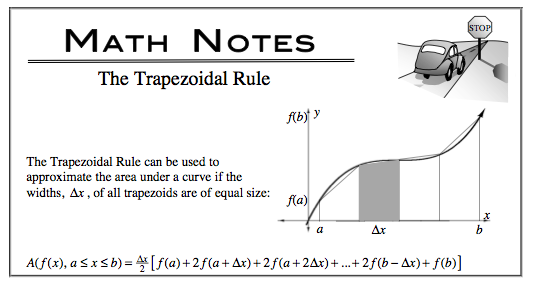### Home > CALC > Chapter 3 > Lesson 3.3.3 > Problem3-121

3-121.Notice the units. Time is given in seconds, but velocity is given in miles per hour.

Distance traveled can be found by calculating area under a velocity graph. Since we cannot see the graph and do not know the function, we must approximate the area given the select values in the table.

Which do you think will give the most accurate approximation: rectangles or trapezoids?